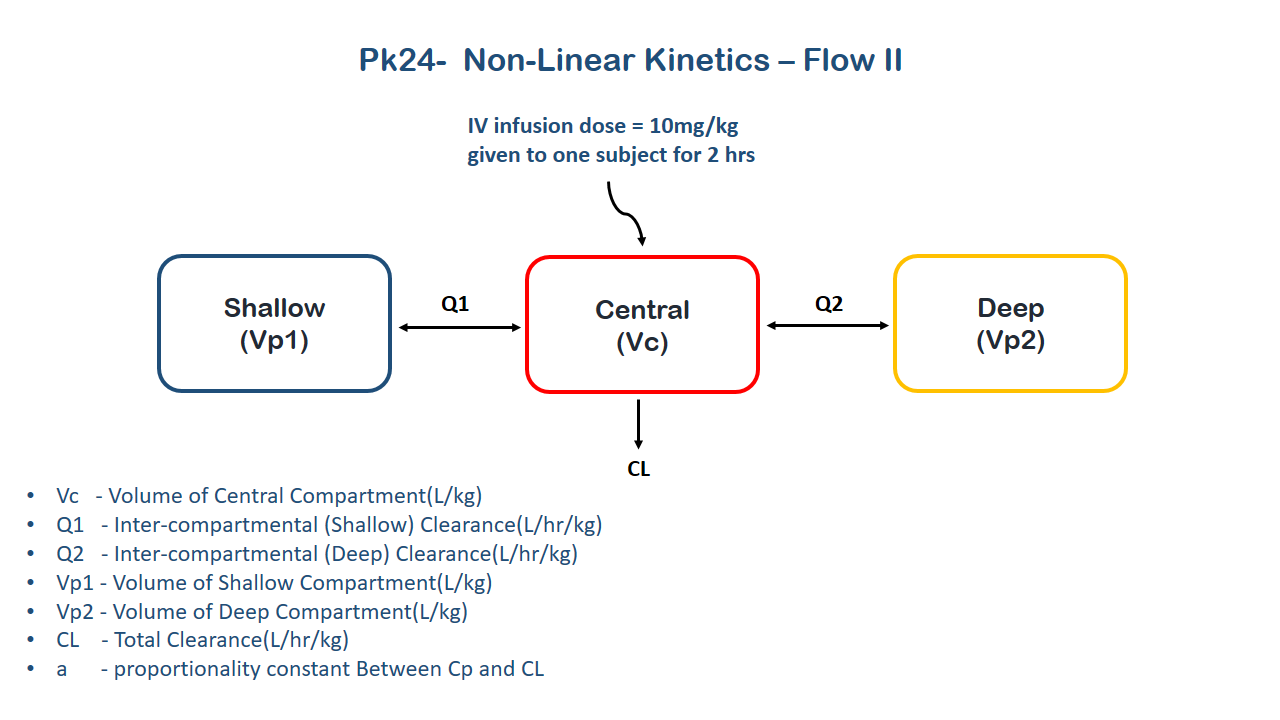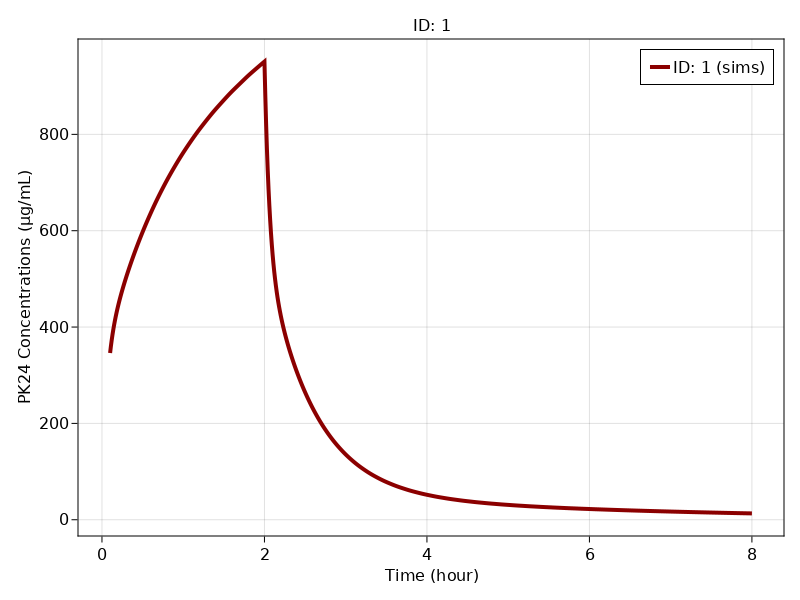# Exercise PK24 - Nonlinear kinetics - Flow 2

### Background

Following info:

• Structural model - Multi-compartment(three) model with concentration dependant clearance

• Route of administration - IV infusion

• Dosage Regimen - One IV infusion dose of 10mg/kg given for 2 hrs.

• Number of Subjects - 1### Learning Outcomes

This exercise explains about the Multi-compartment model following concentration dependant clearance.

### Objectives

In this tutorial you will learn:

• To build a multi-compartment with concentration dependant clearance.

• To simulate the data for one subject given Iv infusion for 2 hrs.

### Libraries

Call the "necessary" libraries to get started.

using Random
using Pumas
using PumasUtilities
using CairoMakie


### Model

This model contains three compartments (Central, shallow and deep) and the clearance is dependant on the change in plasma concentration due to the drug which is known to reduce cardiac output and hepatic blood flow with increase in plasma concentration. The change in clearance can be accounted by the equation: $CL= CLo-A(Central/Vc)$ where $A$ is proportionality constant between $CL$ and $C$

pk_24                = @model begin
desc = "Three Compartment Model"
timeu = u"hr"
end

@param begin
"Volume of Central Compartment(L/kg)"
tvvc        ∈ RealDomain(lower=0)
"Clearance(L/hr/kg)"
tvclo       ∈ RealDomain(lower=0)
"Inter-compartmental (Shallow) Clearance(L/hr/kg)"
tvq1        ∈ RealDomain(lower=0)
"Inter-compartmental (Deep) Clearance(L/hr/kg)"
tvq2        ∈ RealDomain(lower=0)
"Volume of Shallow Compartment(L/kg)"
tvvp1       ∈ RealDomain(lower=0)
"Volume of Deep Compartment(L/kg)"
tvvp2       ∈ RealDomain(lower=0)
"Proportionality constant Between Cp and CL(L2/hr/μg/kg)"
tva         ∈ RealDomain(lower=0)
Ω           ∈ PDiagDomain(7)
σ_prop      ∈ RealDomain(lower=0)
end

@random begin
η           ~ MvNormal(Ω)
end

@pre begin
Vc          = tvvc*exp(η)
CLo         = tvclo*exp(η)
Q1          = tvq1*exp(η)
Q2          = tvq2*exp(η)
Vp1         = tvvp1*exp(η)
Vp2         = tvvp2*exp(η)
A           = 1000*tva*exp(η)
end

@dynamics begin
Central'    =  - (CLo -(A * (Central/Vc))) * (Central/Vc) - Q1*(Central/Vc) + Q1*(Shallow/Vp1) - Q2*(Central/Vc) + Q2*(Deep/Vp2)
Shallow'    =    Q1*(Central/Vc) - Q1*(Shallow/Vp1)
Deep'       =    Q2*(Central/Vc) - Q2*(Deep/Vp2)
end

@derived begin
cp         = @. 1000*Central/Vc
"""
Observed Concentration (ug/L)
"""
dv         ~ @. Normal(cp, sqrt(cp^2*σ_prop))
end
end

PumasModel
Parameters: tvvc, tvclo, tvq1, tvq2, tvvp1, tvvp2, tva, Ω, σ_prop
Random effects: η
Covariates:
Dynamical variables: Central, Shallow, Deep
Derived: cp, dv
Observed: cp, dv


### Parameters

The parameters are as given below. tv represents the typical value for parameters.

• $Vc$ - Volume of Central Compartment(L/kg)

• $CLo$ - Clearance(L/hr/kg)

• $Q1$ - Inter-compartmental (Shallow) Clearance(L/hr/kg)

• $Q2$ - Inter-compartmental (Deep) Clearance(L/hr/kg)

• $Vp1$ - Volume of Shallow Compartment(L/kg)

• $Vp2$ - Volume of Deep Compartment(L/kg)

• $A$ - proportionality constant Between Cp and CL(L2/hr/μg/kg)

• $Ω$ - Between Subject Variability

• $σ$ - Residual error (for plasma conc)

param = ( tvvc  = 0.68,
tvclo = 6.61,
tvq1  = 5.94,
tvq2  = 0.93,
tvvp1 = 1.77,
tvvp2 = 3.20,
tva   = 0.0025,
Ω     = Diagonal([0.0,0.0,0.0,0.0,0.0,0.0,0.0]),
σ_prop= 0.004)

(tvvc = 0.68, tvclo = 6.61, tvq1 = 5.94, tvq2 = 0.93, tvvp1 = 1.77, tvvp2 =
3.2, tva = 0.0025, Ω = [0.0 0.0 … 0.0 0.0; 0.0 0.0 … 0.0 0.0; … ; 0.0 0.0
… 0.0 0.0; 0.0 0.0 … 0.0 0.0], σ_prop = 0.004)


### DosageRegimen

To Build the dosage regimen for one subject given IV infusion of 10mg/kg for 2hrs.

DR = DosageRegimen(10, time = 0, cmt = 1, duration = 2)
s1 = Subject(id = 1, events = DR)

Subject
ID: 1
Events: 2


### Simulation

To simulate the data with specific data points.

sim_sub1 = simobs(pk_24, s1, param, obstimes= 0.1:0.01:8)


### Visualization

f, a, p = sim_plot(pk_24, [sim_sub1],
observations = :cp,
color = :redsblues,
linewidth = 4,
axis = (xlabel = "Time (hour)",
ylabel = "PK24 Concentrations (μg/mL)",
xticks = 0:2:8))
axislegend(a)
f